## 3.6.2 Electron-Phonon Interaction

The electron-phonon interaction HAMILTONian can be written as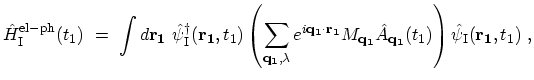(3.47)

where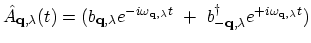,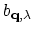and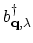are the annihilation and creation operators for phonons with wave-vector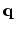, polarization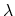, and energy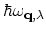, and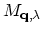is the electron-phonon interaction matrix element. The zero-order perturbation gives the non-interacting GREEN's function. The first-order term of the perturbation expansion must vanish because it contains the factor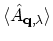which is zero since the factors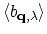and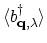are zero . Similarly, all the odd terms vanish because their time-ordered bracket for phonons contains an odd number of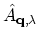factors. Applying the WICK theorem (Section 3.4.1), only the even terms contribute to the perturbation expansion for the electron-phonon interaction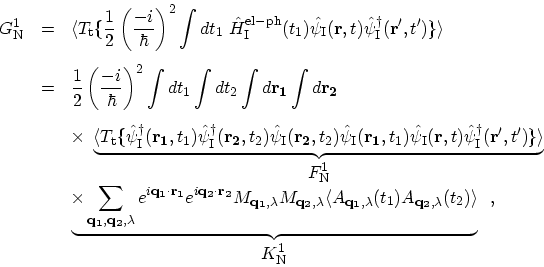(3.48)

where the expansion of time-ordered products of electron operators (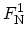) has been calculated before, see (3.37). Notice that, due to the properties of the annihilation and creation operators for Bosons ,unless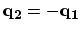, therefore, one obtains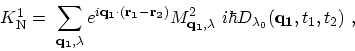(3.49)

where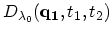is the non-interacting phonon GREEN's function (see Appendix D). FEYNMAN diagrams for this expansion are similar to Fig. 3.4, but one should only replace the COULOMB interactions with non-interacting phonon GREEN's functions . However, the contributions of the diagrams (a), (b), and (f) are zero. They are non-zero only if the phonon wave-vectoris zero, but such phonon is either a translation of the crystal or a permanent strain, and neither of these meant to be in the HAMILTONian. The lowest order self-energies due to electron-phonon interaction are also referred to as HARTREE and FOCK self-energy by analogy to the treatment of the electron-electron interaction. However, the HARTREE self-energy due to electron-phonon interaction is zero since it corresponds to phonons with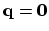. The analytical expression regarding the contribution of the self-consistent FOCK self-energy (Fig. 3.9) is given by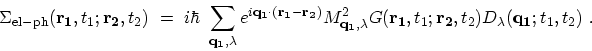(3.50)

M. Pourfath: Numerical Study of Quantum Transport in Carbon Nanotube-Based Transistors